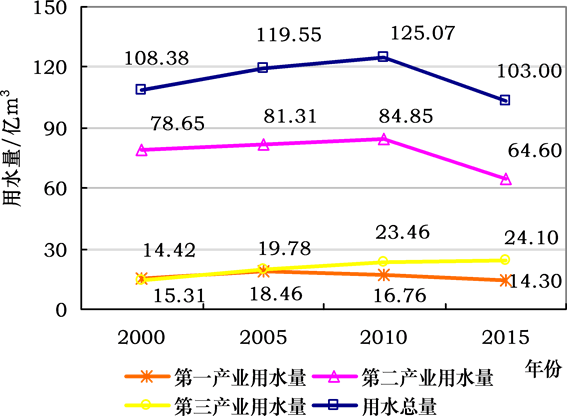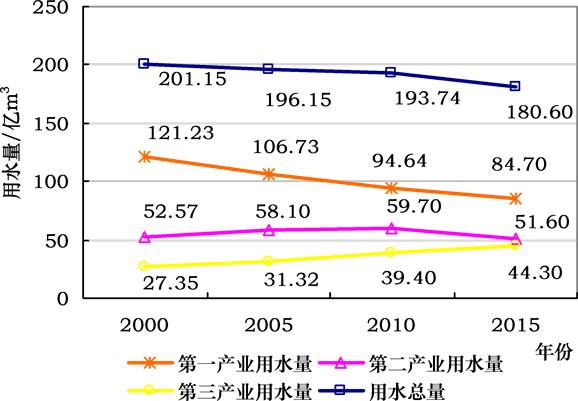﻿ 基于完全分解法的长江三角洲地区用水量时空分异研究

# 基于完全分解法的长江三角洲地区用水量时空分异研究Spatial-Temporal Differences in Water Use in the Yangtze River Delta Based on Complete Decomposition Method

Abstract: Based on the complete decomposition method, this paper decomposes the spatio-temporal dif-ferences in water use in the Yangtze River Delta from 2000 to 2015 into population effect, income effect, structural effect and intensity effect. The results show that: 1) Economic growth and expan-sion of population size are main factors to promote the increase of water use, while the decline in industrial water intensity and upgrading of industrial structure are the factors that inhibit the in-crease in water use; 2) The water use efficiency in the three industries in the Yangtze River Delta has generally risen, which has effectively promoted the decline in water use, especially the sec-ondary industry. The role of industrial restructuring mainly comes from primary industry; 3) The main reasons for the higher water use in Jiangsu and Zhejiang than Shanghai are the large popula-tion size and low industrial structure. Therefore, to achieve a reduction in water use, we should further reduce industrial water intensity, optimize and upgrade the industrial structure, of course, still need focus on different industries; weighing the relationship between economic growth and water use, and controlling population size are also important ways to reduce water consumption; Jiangsu and Zhejiang can reduce the difference between water use and Shanghai by controlling population size and optimizing and upgrading industrial structure.

1. 引言

2. 模型构建

$W=\underset{i=1}{\overset{3}{\sum }}{W}_{i}=\underset{i=1}{\overset{3}{\sum }}P×\frac{G}{P}×\frac{{G}_{i}}{G}×\frac{{W}_{i}}{{G}_{i}}$ (1)

$G=\underset{i=1}{\overset{3}{\sum }}{W}_{i}$ ( $i=1,2,3$ )。

$W=\underset{i=1}{\overset{3}{\sum }}P×Inc×{S}_{i}×{I}_{i}$ (2)

$\Delta W={W}^{t}-{W}^{0}=\underset{i=1}{\overset{3}{\sum }}{P}^{t}×In{c}^{t}×{S}_{i}^{t}×{I}_{i}^{t}-\underset{i=1}{\overset{3}{\sum }}{P}^{0}×In{c}^{0}×{S}_{i}^{0}×{I}_{i}^{0}$ (3)

$\Delta W=\Delta {W}_{P}+\Delta {W}_{Inc}+\Delta {W}_{S}+\Delta {W}_{I}$ (4)

$\begin{array}{l}\Delta {W}_{P}=\underset{i=1}{\overset{3}{\sum }}\Delta P\cdot In{c}^{0}\cdot {S}_{i}^{0}\cdot {I}_{i}^{0}+\frac{1}{2}\underset{i=1}{\overset{3}{\sum }}\Delta P\cdot \left(\Delta Inc\cdot {S}_{i}^{0}\cdot {I}_{i}^{0}+\Delta {S}_{i}\cdot In{c}^{0}\cdot {I}_{i}^{0}+\Delta {I}_{i}^{0}\cdot In{c}^{0}\cdot {S}_{i}^{0}\right)\\ \text{ }\text{ }\text{ }\text{ }\text{ }\text{ }\text{ }\text{ }\text{ }\text{ }\text{ }\text{ }\text{ }\text{ }\text{ }\text{ }\text{ }\text{ }\text{ }\text{ }\text{ }\text{\hspace{0.17em}}\text{\hspace{0.17em}}\text{ }+\frac{1}{3}\underset{i=1}{\overset{3}{\sum }}\Delta P\cdot \left(\Delta Inc\cdot \Delta {S}_{i}\cdot {I}_{i}^{0}+\Delta Inc\cdot \Delta {I}_{i}\cdot {S}_{i}^{0}+\Delta {S}_{i}\cdot \Delta {I}_{i}\cdot In{c}^{0}\right)\\ \text{\hspace{0.17em}}\text{\hspace{0.17em}}\text{\hspace{0.17em}}\text{\hspace{0.17em}}\text{\hspace{0.17em}}\text{\hspace{0.17em}}\text{\hspace{0.17em}}\text{\hspace{0.17em}}\text{\hspace{0.17em}}+\frac{1}{4}\underset{i=1}{\overset{3}{\sum }}\Delta P\cdot \left(\Delta Inc\cdot \Delta {S}_{i}\cdot \Delta {I}_{i}\right)\end{array}$ (5)

$\begin{array}{l}\Delta {W}_{Inc}=\underset{i=1}{\overset{3}{\sum }}\Delta Inc\cdot {P}^{0}\cdot {S}_{i}{}^{0}\cdot {I}_{i}^{0}+\frac{1}{2}\underset{i=1}{\overset{3}{\sum }}\Delta Inc\cdot \left(\Delta P\cdot {S}_{i}^{0}\cdot {I}_{i}^{0}+\Delta {S}_{i}\cdot {P}^{0}\cdot {I}_{i}^{0}+\Delta {I}_{i}\cdot {P}^{0}\cdot {S}_{i}^{0}\right)\\ \text{ }\text{ }\text{ }\text{ }\text{ }\text{ }\text{ }\text{ }\text{ }\text{ }\text{ }\text{ }\text{ }\text{ }\text{ }\text{ }\text{ }\text{ }\text{ }\text{\hspace{0.17em}}\text{\hspace{0.17em}}\text{\hspace{0.17em}}\text{\hspace{0.17em}}\text{ }\text{ }+\frac{1}{3}\underset{i=1}{\overset{3}{\sum }}\Delta Inc\cdot \left(\Delta P\cdot \Delta {S}_{i}\cdot {I}_{i}^{0}+\Delta P\cdot \Delta {I}_{i}\cdot {S}_{i}^{0}+\Delta {S}_{i}\cdot \Delta {I}_{i}\cdot {P}^{0}\right)\\ \text{\hspace{0.17em}}\text{\hspace{0.17em}}\text{\hspace{0.17em}}\text{\hspace{0.17em}}\text{\hspace{0.17em}}\text{\hspace{0.17em}}\text{\hspace{0.17em}}\text{\hspace{0.17em}}\text{\hspace{0.17em}}\text{\hspace{0.17em}}\text{\hspace{0.17em}}+\frac{1}{4}\underset{i=1}{\overset{3}{\sum }}\Delta Inc\cdot \left(\Delta P\cdot \Delta {S}_{i}\cdot \Delta {I}_{i}\right)\end{array}$ (6)

$\begin{array}{l}\Delta {W}_{S}=\underset{i=1}{\overset{3}{\sum }}\Delta {S}_{i}\cdot {P}^{0}\cdot In{c}^{0}\cdot {I}_{i}^{0}+\frac{1}{2}\underset{i=1}{\overset{3}{\sum }}\Delta {S}_{i}\cdot \left(\Delta P\cdot In{c}^{0}\cdot {I}_{i}^{0}+\Delta Inc\cdot {P}^{0}\cdot {I}_{i}^{0}+\Delta {I}_{i}\cdot {P}^{0}\cdot In{c}^{0}\right)\\ \text{ }\text{ }\text{ }\text{ }\text{ }\text{ }\text{ }\text{ }\text{ }\text{ }\text{ }\text{ }\text{ }\text{ }\text{ }\text{\hspace{0.17em}}\text{\hspace{0.17em}}\text{ }\text{ }\text{ }\text{ }\text{ }\text{ }\text{ }+\frac{1}{3}\underset{i=1}{\overset{3}{\sum }}\Delta {S}_{i}\cdot \left(\Delta P\cdot \Delta Inc\cdot {I}_{i}^{0}+\Delta P\cdot \Delta {I}_{i}\cdot In{c}^{0}+\Delta Inc\cdot \Delta {I}_{i}\cdot {P}^{0}\right)\\ \text{\hspace{0.17em}}\text{\hspace{0.17em}}\text{\hspace{0.17em}}\text{\hspace{0.17em}}\text{\hspace{0.17em}}\text{\hspace{0.17em}}\text{\hspace{0.17em}}\text{\hspace{0.17em}}\text{\hspace{0.17em}}+\frac{1}{4}\underset{i=1}{\overset{3}{\sum }}\Delta {S}_{i}\cdot \left(\Delta P\cdot \Delta Inc\cdot \Delta {I}_{i}\right)\end{array}$ (7)

$\begin{array}{l}\Delta {W}_{I}=\underset{i=1}{\overset{3}{\sum }}\Delta {I}_{i}\cdot {P}^{0}\cdot In{c}^{0}\cdot {S}_{i}^{0}+\frac{1}{2}\underset{i=1}{\overset{3}{\sum }}\Delta {I}_{i}\cdot \left(\Delta P\cdot In{c}^{0}\cdot {S}_{i}^{0}+\Delta Inc\cdot {P}^{0}\cdot {S}_{i}^{0}+\Delta {S}_{i}\cdot {P}^{0}\cdot In{c}^{0}\right)\\ \text{ }\text{ }\text{ }\text{ }\text{ }\text{ }\text{ }\text{ }\text{ }\text{ }\text{ }\text{ }\text{ }\text{ }\text{ }\text{ }\text{ }\text{ }\text{ }\text{ }\text{ }\text{\hspace{0.17em}}\text{\hspace{0.17em}}+\frac{1}{3}\underset{i=1}{\overset{3}{\sum }}\Delta {I}_{i}\cdot \left(\Delta P\cdot \Delta Inc\cdot {S}_{i}^{0}+\Delta P\cdot \Delta {S}_{i}\cdot In{c}^{0}+\Delta Inc\cdot \Delta {S}_{i}\cdot {P}^{0}\right)\\ \text{\hspace{0.17em}}\text{\hspace{0.17em}}\text{\hspace{0.17em}}\text{\hspace{0.17em}}\text{\hspace{0.17em}}\text{\hspace{0.17em}}\text{\hspace{0.17em}}\text{\hspace{0.17em}}+\frac{1}{4}\underset{i=1}{\overset{3}{\sum }}\Delta {I}_{i}\cdot \left(\Delta P\cdot \Delta Inc\cdot \Delta {S}_{i}\right)\end{array}$ (8)

$\Delta W={W}^{R1}-{W}^{R0}=\underset{i=1}{\overset{3}{\sum }}{P}^{R1}×In{c}^{R1}×{S}_{i}^{R1}×{I}_{i}^{R1}-\underset{i=1}{\overset{3}{\sum }}{P}^{R0}×In{c}^{R0}×{S}_{i}^{R0}×{I}_{i}^{R0}$ (9)

$\Delta {W}^{R1-R0}=\Delta {W}_{P}^{R1-R0}+\Delta {W}_{Inc}^{R1-R0}+\Delta {W}_{S}^{R1-R0}+\Delta {W}_{I}^{R1-R0}$ (10)

$\begin{array}{l}\Delta {W}_{P}^{R1-R0}=\underset{i=1}{\overset{3}{\sum }}\Delta P\cdot In{c}^{R0}\cdot {S}_{i}^{R0}\cdot {I}_{i}^{R0}\\ \text{ }\text{ }\text{ }\text{ }\text{ }\text{ }\text{ }\text{ }\text{ }\text{ }\text{ }\text{ }\text{ }\text{ }\text{ }\text{ }\text{ }\text{ }\text{ }\text{ }\text{ }\text{ }\text{ }\text{ }\text{ }\text{ }\text{ }\text{ }\text{ }\text{ }\text{ }\text{ }\text{ }\text{ }\text{ }\text{ }\text{ }\text{ }\text{\hspace{0.17em}}\text{\hspace{0.17em}}+\frac{1}{2}\underset{i=1}{\overset{3}{\sum }}\Delta P\cdot \left(\Delta Inc\cdot {S}_{i}^{R0}\cdot {I}_{i}^{R0}+\Delta {S}_{i}\cdot In{c}^{R0}\cdot {I}_{i}^{R0}+\Delta {I}_{i}\cdot In{c}^{R0}\cdot {S}_{i}^{R0}\right)\\ \text{ }\text{ }\text{ }\text{ }\text{ }\text{ }\text{ }\text{ }\text{ }\text{ }\text{ }\text{ }\text{ }\text{ }\text{ }\text{ }\text{ }\text{ }\text{ }\text{ }\text{ }\text{ }\text{ }\text{ }\text{ }\text{ }\text{ }\text{ }\text{ }\text{ }\text{\hspace{0.17em}}\text{\hspace{0.17em}}\text{ }\text{ }\text{ }\text{ }\text{ }\text{ }\text{ }\text{ }+\frac{1}{3}\underset{i=1}{\overset{3}{\sum }}\Delta P\cdot \left(\Delta Inc\cdot \Delta {S}_{i}\cdot {I}_{i}^{R0}+\Delta Inc\cdot \Delta {I}_{i}\cdot {S}_{i}^{R0}+\Delta {S}_{i}\cdot \Delta {I}_{i}\cdot In{c}^{R0}\right)\text{ }\\ \text{ }\text{ }\text{ }\text{ }\text{ }\text{ }\text{ }\text{ }\text{ }\text{ }\text{ }\text{ }\text{ }\text{ }\text{ }\text{ }\text{ }\text{ }\text{ }\text{ }\text{ }\text{ }\text{ }\text{ }\text{ }\text{ }\text{\hspace{0.17em}}\text{\hspace{0.17em}}\text{ }\text{ }\text{ }\text{ }\text{ }\text{ }\text{ }\text{ }\text{ }\text{ }\text{ }\text{ }+\frac{1}{4}\underset{i=1}{\overset{3}{\sum }}\Delta P\cdot \left(\Delta Inc\cdot \Delta {S}_{i}\cdot \Delta {I}_{i}\right)\end{array}$ (11)

$\begin{array}{l}\Delta {W}_{Inc}^{R1-R0}=\underset{i=1}{\overset{3}{\sum }}\Delta Inc\cdot {P}^{R0}\cdot {S}_{i}^{R0}\cdot {I}_{i}^{R0}\\ \text{ }\text{ }\text{ }\text{ }\text{ }\text{ }\text{ }\text{ }\text{ }\text{ }\text{ }\text{ }\text{ }\text{ }\text{ }\text{ }\text{ }\text{ }\text{ }\text{ }\text{ }\text{ }\text{ }\text{ }\text{ }\text{ }\text{ }\text{ }\text{ }\text{ }\text{ }\text{ }\text{ }\text{\hspace{0.17em}}\text{ }\text{ }\text{ }\text{ }\text{ }\text{ }\text{ }\text{ }+\frac{1}{2}\underset{i=1}{\overset{3}{\sum }}\Delta Inc\cdot \left(\Delta P\cdot {S}_{i}^{R0}\cdot {I}_{i}^{R0}+\Delta {S}_{i}\cdot {P}^{R0}\cdot {I}_{i}^{R0}+\Delta {I}_{i}\cdot {P}^{R0}\cdot {S}_{i}^{R0}\right)\\ \text{ }\text{ }\text{ }\text{ }\text{ }\text{ }\text{ }\text{ }\text{ }\text{ }\text{ }\text{ }\text{ }\text{ }\text{ }\text{ }\text{ }\text{ }\text{ }\text{ }\text{ }\text{ }\text{ }\text{ }\text{ }\text{ }\text{ }\text{\hspace{0.17em}}\text{ }\text{ }\text{ }\text{ }\text{ }\text{ }\text{ }\text{ }\text{ }\text{ }\text{ }\text{ }\text{ }\text{ }+\frac{1}{3}\underset{i=1}{\overset{3}{\sum }}\Delta Inc\cdot \left(\Delta P\cdot \Delta {S}_{i}\cdot {I}_{i}^{R0}+\Delta P\cdot \Delta {I}_{i}\cdot {S}_{i}^{R0}+\Delta {S}_{i}\cdot \Delta {I}_{i}\cdot {P}^{R0}\right)\\ \text{ }\text{ }\text{ }\text{ }\text{ }\text{ }\text{ }\text{ }\text{ }\text{ }\text{ }\text{ }\text{ }\text{ }\text{ }\text{ }\text{ }\text{ }\text{ }\text{ }\text{ }\text{ }\text{ }\text{ }\text{ }\text{ }\text{ }\text{\hspace{0.17em}}\text{ }\text{ }\text{ }\text{ }\text{ }\text{ }\text{ }\text{ }\text{ }\text{ }\text{ }\text{ }\text{ }\text{ }+\frac{1}{4}\underset{i=1}{\overset{3}{\sum }}\Delta Inc\cdot \left(\Delta P\cdot \Delta {S}_{i}\cdot \Delta {I}_{i}\right)\end{array}$ (12)

$\begin{array}{l}\Delta {W}_{S}^{R1-R0}=\underset{i=1}{\overset{3}{\sum }}\Delta {S}_{i}\cdot {P}^{R0}\cdot In{c}^{R0}\cdot {I}_{i}^{R0}\\ \text{ }\text{ }\text{ }\text{ }\text{ }\text{ }\text{ }\text{ }\text{ }\text{ }\text{ }\text{ }\text{ }\text{ }\text{ }\text{ }\text{ }\text{ }\text{ }\text{ }\text{ }\text{ }\text{ }\text{ }\text{ }\text{ }\text{ }\text{ }\text{ }\text{ }\text{ }\text{ }\text{ }\text{ }\text{\hspace{0.17em}}\text{\hspace{0.17em}}\text{ }\text{ }\text{ }\text{ }\text{ }+\frac{1}{2}\underset{i=1}{\overset{3}{\sum }}\Delta {S}_{i}\cdot \left(\Delta P\cdot In{c}^{R0}\cdot {I}_{i}^{R0}+\Delta Inc\cdot {P}^{R0}\cdot {I}_{i}^{R0}+\Delta {I}_{i}\cdot {P}^{R0}\cdot In{c}^{R0}\right)\\ \text{ }\text{ }\text{ }\text{ }\text{ }\text{ }\text{ }\text{ }\text{ }\text{ }\text{ }\text{ }\text{ }\text{ }\text{ }\text{ }\text{ }\text{ }\text{ }\text{ }\text{ }\text{ }\text{ }\text{ }\text{ }\text{ }\text{ }\text{\hspace{0.17em}}\text{ }\text{ }\text{ }\text{ }\text{ }\text{ }\text{ }\text{\hspace{0.17em}}\text{ }\text{ }\text{ }\text{ }\text{ }+\frac{1}{3}\underset{i=1}{\overset{3}{\sum }}\Delta {S}_{i}\cdot \left(\Delta P\cdot \Delta Inc\cdot {I}_{i}^{R0}+\Delta P\cdot \Delta {I}_{i}\cdot In{c}^{R0}+\Delta Inc\cdot \Delta {I}_{i}\cdot {P}^{R0}\right)\\ \text{ }\text{ }\text{ }\text{ }\text{ }\text{ }\text{ }\text{ }\text{ }\text{ }\text{ }\text{ }\text{ }\text{ }\text{ }\text{ }\text{ }\text{ }\text{ }\text{ }\text{ }\text{ }\text{ }\text{ }\text{ }\text{ }\text{ }\text{ }\text{\hspace{0.17em}}\text{\hspace{0.17em}}\text{ }\text{ }\text{ }\text{ }\text{ }\text{ }\text{ }\text{ }\text{ }\text{ }\text{ }+\frac{1}{4}\underset{i=1}{\overset{3}{\sum }}\Delta {S}_{i}\cdot \left(\Delta P\cdot \Delta Inc\cdot \Delta {I}_{i}\right)\end{array}$ (13)

$\begin{array}{l}\Delta {W}_{I}^{R1-R0}=\underset{i=1}{\overset{3}{\sum }}\Delta {I}_{i}\cdot {P}^{R0}\cdot In{c}^{R0}\cdot {S}_{i}^{R0}\\ \text{ }\text{ }\text{ }\text{ }\text{ }\text{ }\text{ }\text{ }\text{ }\text{ }\text{ }\text{ }\text{ }\text{ }\text{ }\text{ }\text{ }\text{ }\text{ }\text{ }\text{ }\text{ }\text{ }\text{ }\text{ }\text{ }\text{ }\text{ }\text{ }\text{ }\text{ }\text{ }\text{\hspace{0.17em}}\text{ }\text{ }\text{\hspace{0.17em}}\text{ }\text{ }\text{ }\text{ }+\frac{1}{2}\underset{i=1}{\overset{3}{\sum }}\Delta {I}_{i}\cdot \left(\Delta P\cdot In{c}^{R0}\cdot {S}_{i}^{R0}+\Delta Inc\cdot {P}^{R0}\cdot {S}_{i}^{R0}+\Delta {S}_{i}\cdot {P}^{R0}\cdot In{c}^{R0}\right)\\ \text{ }\text{ }\text{ }\text{ }\text{ }\text{ }\text{ }\text{ }\text{ }\text{ }\text{ }\text{ }\text{ }\text{ }\text{ }\text{ }\text{ }\text{ }\text{ }\text{ }\text{ }\text{ }\text{ }\text{ }\text{ }\text{ }\text{ }\text{ }\text{ }\text{ }\text{ }\text{\hspace{0.17em}}\text{ }\text{ }\text{\hspace{0.17em}}\text{ }\text{ }\text{ }\text{ }\text{ }+\frac{1}{3}\underset{i=1}{\overset{3}{\sum }}\Delta {I}_{i}\cdot \left(\Delta P\cdot \Delta Inc\cdot {S}_{i}^{R0}+\Delta P\cdot \Delta {S}_{i}\cdot In{c}^{R0}+\Delta Inc\cdot \Delta {S}_{i}\cdot {P}^{R0}\right)\\ \text{ }\text{ }\text{ }\text{ }\text{ }\text{ }\text{ }\text{ }\text{ }\text{ }\text{ }\text{ }\text{ }\text{ }\text{ }\text{ }\text{ }\text{ }\text{ }\text{ }\text{ }\text{ }\text{ }\text{ }\text{ }\text{ }\text{ }\text{ }\text{\hspace{0.17em}}\text{\hspace{0.17em}}\text{ }\text{ }\text{ }\text{ }\text{ }\text{ }\text{ }\text{ }\text{ }\text{ }+\frac{1}{4}\underset{i=1}{\overset{3}{\sum }}\Delta {I}_{i}\cdot \left(\Delta P\cdot \Delta Inc\cdot \Delta {S}_{i}\right)\end{array}$ (14)

3. 数据来源

1) 人口指标。人口指标分为常住人口和户籍人口两种口径，经济发展带动了大规模的人口流动，但是户籍却未发生变化，因此，常住人口指标更能反映一个地区的人口状况。

2) 增加值指标。三次产业增加值均根据2000年不变价格进行调整，以便消除价格因素影响，国内生产总值由调整后的三次产业增加值加总得到，人均国内生产总值由国内生产总值除以人口指标得到。

3) 用水量指标。《中国水资源公报》将省际用水总量划分为农业用水、工业用水、生活用水和生态用水，并不是按照三次产业口径进行划分，为了用水量口径与三次产业口径相一致，因此，需要对用水量分类进行调整，过程如下：将农业用水作为第一产业用水，由于建筑业用水所占比重较小，工业用水作为第二产业用水，同时，参考孙才志  的处理方法，将生活用水作为第三产业用水，用水总量由三次产业用水加总得到，产业用水强度由产业用水量除以产业增加值计算得到。

4. 实证分析

4.1. 长江三角洲地区用水量变化趋势

1) 从时间视角来看，江苏用水总量从2000年的445.60亿m3增加到2005年的514.76亿m3，从2010年的548.99亿m3增加到2015年的572.50亿m3，2000~2015年16年期间，年均增长率为1.68%，其中，第一产业用水量所占比重最大，4个年份都在40%以上，2000年将近60%，第二产业用水量次之，第三产业用水量最小。Figure 1. The change of water use for Shanghai from 2000 to 2015Figure 2. The change of water use for Jiangsu from 2000 to 2015

2) 从空间视角来看，江苏用水总量在4个年份最大，浙江次之，上海最小。其中，三次产业用水量都是江苏最大，第一、三产业用水量浙江次之，上海最小，而第二产业却是上海次之，浙江最小。Figure 3. The change of water use for Zhejiang from 2000 to 2015

4.2. 长江三角洲地区用水量时间差异的效应分解

1) 在2000~2015年期间，江苏用水总量累计增加了126.90亿m3，其中，收入效应 $\Delta {W}_{Inc}$ 和人口效应 $\Delta {W}_{P}$ 分别达到875.53亿m3和45.70亿m3，前者是后者的19倍，表明经济增长和人口规模扩大是推动用水总量增加的主要和次要因素。强度效应 $\Delta {W}_{I}$ 和结构效应 $\Delta {W}_{S}$ 分别达到−479.95亿m3和−314.38亿m3，前者是后者的1.5倍，表明产业用水强度下降和产业结构优化升级是抑制用水总量增加的主要和次要因素。

2) 浙江在考察期内用水总量累计下降了20.55亿m3，其中，收入效应 $\Delta {W}_{Inc}$ 和人口效应 $\Delta {W}_{P}$ 分别达到281.07亿m3和34.32亿m3，前者是后者的8倍，表明经济增长和人口规模扩大是推动用水总量增加的主要和次要因素。强度效应 $\Delta {W}_{I}$ 和结构效应 $\Delta {W}_{S}$ 分别达到−223.10亿m3和−112.84亿m3，前者是后者的2倍，表明产业用水强度下降和产业结构优化升级是抑制用水总量的主要和次要因素。三个规划期用水总量分别下降了5.00亿m3、2.41亿m3和13.14亿m3。收入效应 $\Delta {W}_{Inc}$ 和人口效应 $\Delta {W}_{P}$ 都是正值，表明经济增长和人口规模扩大始终是用水量增加的推动因素，尤其是前者。强度效应 $\Delta {W}_{I}$ 和结构效应 $\Delta {W}_{S}$ 始终是负值，表明产业用水强度下降和产业结构优化升级是用水量增加的抑制因素，尤其是前者。与江苏不同的是，产业用水强度下降和产业结构优化升级对用水量的促减作用大于经济增长和人口规模扩大Table 1. The driving effect decomposition results of water use in Yangtze River Delta from 2000 to 2015

3) 上海在考察期内用水总量累计下降了5.38亿m3，其中，收入效应 $\Delta {W}_{Inc}$ 和人口效应 $\Delta {W}_{P}$ 分别达到124.88亿m3和49.51亿m3，前者是后者的2.5倍。表明经济增长和人口规模扩大是推动用水总量增加的主要和次要因素。强度效应 $\Delta {W}_{I}$ 和结构效应 $\Delta {W}_{S}$ 分别达到−142.01亿m3和−37.77亿m3，前者是后者的3.8倍，表明产业用水强度下降和产业结构优化升级是抑制用水总量的主要和次要因素。三个规划期用水总量变化量分别为11.17亿m3、5.52亿m3和−22.07亿m3。收入效应 $\Delta {W}_{Inc}$ 和人口效应 $\Delta {W}_{P}$ 都是正值，表明经济增长和人口规模扩大始终是用水量增加的推动因素，尤其是前者。强度效应 $\Delta {W}_{I}$ 和结构效应 $\Delta {W}_{S}$ 始终是负值，表明产业用水强度下降和产业结构优化升级是用水量增加的抑制因素，尤其是前者。Table 2. The industrial difference of driving effect of water use in Yangtze River Delta

4.3. 长江三角洲地区用水量空间差异的效应分解

1) 江苏与上海用水总量空间差异的驱动效应分解：在2000年、2005年、2010年和2015年，江苏用水总量比上海分别多337.22亿m3、395.21亿m3、423.92亿m3和469.50亿m3，差距逐渐扩大。其中，人口效应 $\Delta {W}_{P}^{R1-R0}$ 和结构效应 $\Delta {W}_{S}^{R1-R0}$ 始终是正值，表明江苏与上海相比，人口规模更大，产业结构比较低级，是江苏用水总量大于上海的推动因素。收入效应 $\Delta {W}_{Inc}^{R1-R0}$ 始终是负值，表明江苏与上海相比，人均GDP较小，抑制了江苏用水总量与上海的差距拉大，不过其绝对值呈递减特征，说明两个地区经济发展水平差异带动用水总量差异的作用逐渐缩小。强度效应 $\Delta {W}_{I}^{R1-R0}$ 在2000年和2005年为正值，在2010年和2015年为负值，表明江苏产业用水强度由高于上海转变为低于上海，有利于缩小两个地区用水总量的差异。

2) 浙江与上海用水总量空间差异的驱动效应分解：在2000年、2005年、2010年和2015年，浙江用水总量分别比上海多92.77亿m3、76.60亿m3、68.67亿m3和77.60亿m3。其中，人口效应 $\Delta {W}_{P}^{R1-R0}$ 和结构效应 $\Delta {W}_{S}^{R1-R0}$ 是正值，表明浙江与上海相比，人口规模更大，产业结构比较低级，是浙江用水总量大于上海的推动因素。收入效应 $\Delta {W}_{Inc}^{R1-R0}$ 和强度效应 $\Delta {W}_{I}^{R1-R0}$ 始终是负值，表明浙江与上海相比，人均GDP较小，产业用水强度更低，对浙江用水总量大于上海起到抑制作用。

5. 结论与建议

1) 2000~2015年以及子时间段内，经济增长( $\Delta {W}_{Inc}$ )和人口规模扩大( $\Delta {W}_{P}$ )是推动长江三角洲地区用Table 3. Decomposition effect of water spatial difference between Jiangsu and ShanghaiTable 4. Decomposition effect of water spatial difference between Zhejiang and Shanghai

1) 进一步降低产业用水强度，优化升级产业结构来实现用水量下降；正确权衡经济增长与水资源消耗、经济增长的数量与质量之间的关系；控制人口规模也是减少用水量的重要途径。2) 针对不同产业，用水效率提高策略应该有所侧重，应该重点放在第二、一产业，第三产业用水效率提升空间有限；优化产业结构主要在于降低第一产业比重。3) 与上海相比，江苏、浙江缩小用水总量差异，可以通过控制人口规模，优化升级产业结构(由高耗水的第一产业向低耗水的第二、三产业转移)来实现。

 本书编写组. 《国务院关于实行最严格水资源管理制度的意见》辅导读本[M]. 北京: 中国水利水电出版社, 2012.

 陈东景. 中国工业水资源消耗强度变化的结构份额和效率份额研究[J]. 中国人口.资源与环境, 2008, 18(3): 211-214.

 刘翀, 柏明国. 安徽省工业行业用水消耗变化分析——基于LMDI分解法[J]. 资源科学, 2012, 34(12): 2299-2305.

 张礼兵, 徐勇俊, 金菊良, 等. 安徽省工业用水量变化影响因素分析[J]. 水利学报, 2014, 45(7): 837-843.

 秦昌波, 葛察忠, 贾仰文, 等. 陕西省生产用水变动的驱动机制分析[J]. 中国人口•资源与环境, 2015, 25(5): 131-136.

 孙才志, 谢巍. 中国产业用水变化驱动效应测度及空间分异[J]. 经济地理, 2011, 31(4): 666-672.

 Xu, Y., Huang, K., Yu, Y., et al. (2015) Changes in Water Footprint of Crop Production in Beijing from 1978 to 2012: A Logarithmic Mean Divisia Index Decomposition Analysis. Journal of Cleaner Production, 87, 180-187.
https://doi.org/10.1016/j.jclepro.2014.08.103

 韩琴, 孙才志, 邹玮. 1998-2012年中国省际灰水足迹效率测度与驱动模式分析[J]. 资源科学, 2016, 38(6): 1179-1191.
https://doi.org/10.18402/resci.2016.06.17

 陈东景. 我国工农业水资源使用强度变动的区域因素分解与差异分析[J]. 自然资源学报, 2012, 27(2): 332-343.

 张陈俊, 章恒全, 龚雅云. 中国结构升级、技术进步与水资源消耗——基于改进的LMDI方法[J]. 资源科学, 2014, 36(10): 1993-2002.

 张陈俊, 章恒全, 陈其勇, 等. 中国用水量变化的影响因素分析——基于LMDI方法[J]. 资源科学, 2016, 38(7): 1308-1322.
https://doi.org/10.18402/resci.2016.07.12

 Ang, B.W., Su, B. and Wang, H. (2016) A Spatial-Temporal Decomposition Approach to Performance Assessment in Energy and Emissions. Energy Economics, 60, 112-121.
https://doi.org/10.1016/j.eneco.2016.08.024

 Sun, J.W. (1998) Changes in Energy Consumption and Energy Intensity: A Complete Decomposition Model. Energy Economics, 20, 85-100.
https://doi.org/10.1016/S0140-9883(97)00012-1

 中华人民共和国国家统计局. 中国统计年鉴[M]. 北京: 中国统计出版社, 2001-2016.

 中华人民共和国水利部. 中国水资源公报[M]. 北京: 中国水利水电出版社, 2000-2015.

Top